# Sample Data: NZ Trees

Locations of New Zealand trees without annotations

## Details

Locations of New Zealand trees in the observation region Rectangle[{0, 0}, {153, 95}] feet without annotations.

## Examples

### Basic Examples (1)

 In:=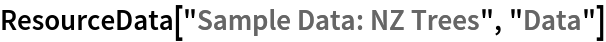Out=Summary of the spatial point data:

 In:=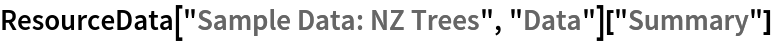Out=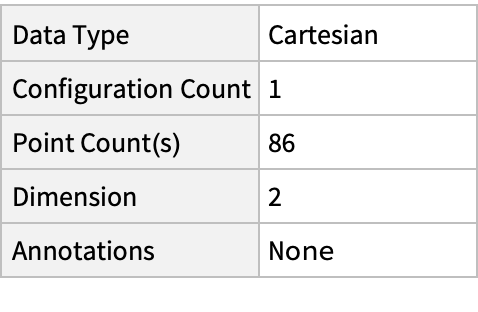### Visualizations (2)

Plot the spatial point data:

 In:=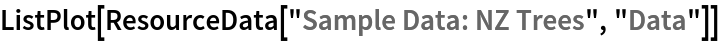Out=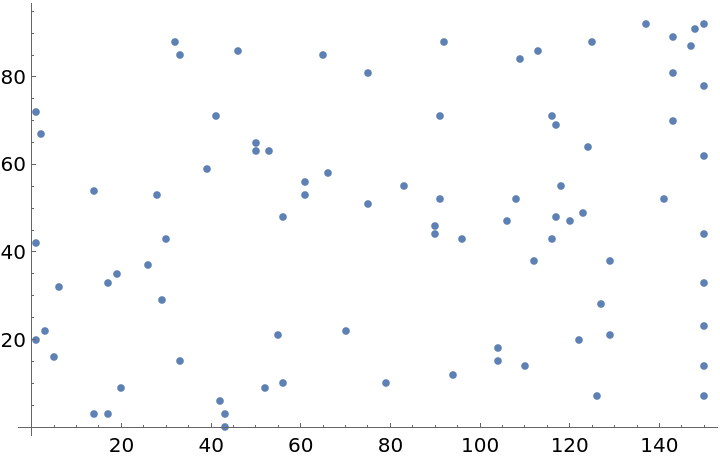Visualize smooth point density of the data:

 In:=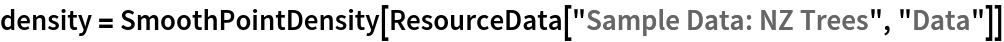Out=In:=Out=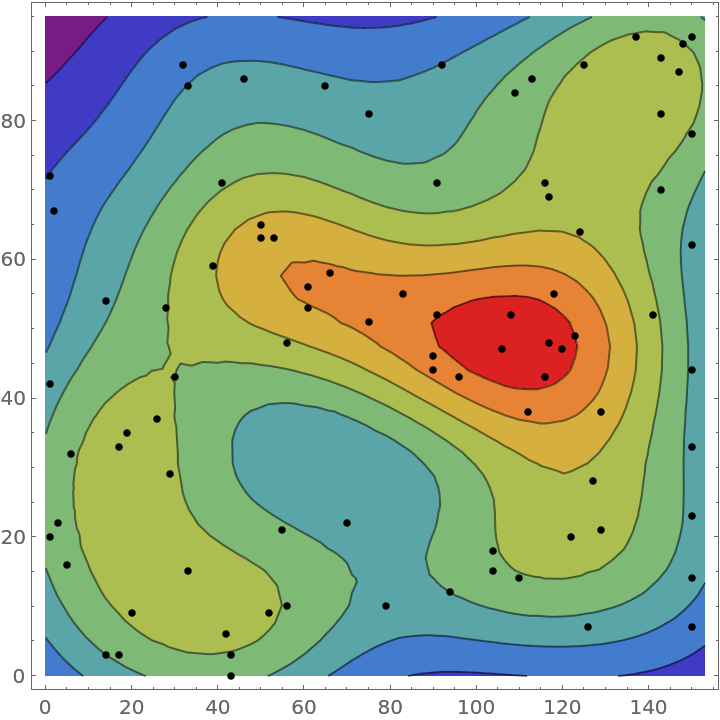### Analysis (6)

Compute probability of finding a point within given radius of an existing point - NearestNeighborG is the CDF of the nearest neighbor distribution:

 In:=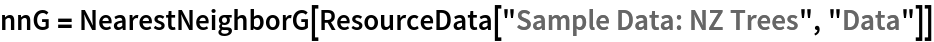Out=In:=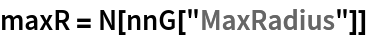Out=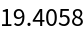In:=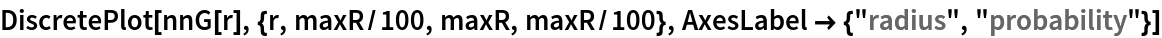Out=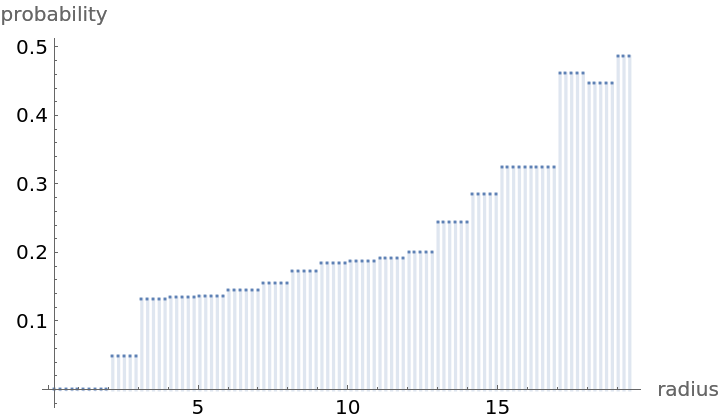NearestNeighborG as the CDF of nearest neighbor distribution can be used to compute the mean distance between a typical point and its nearest neighbor - the mean of a positive support distribution can be approximated via a Riemann sum of 1- CDF. To use Riemann approximation create the partition of the support interval from 0 to maxR into 100 parts and compute the value of the NearestNeighborG at the middle of each subinterval:

 In:=Now compute the Riemann sum to find the mean distance between a typical point and its nearest neighbor:

 In:=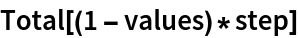Out=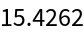Account for scale and units:

 In:=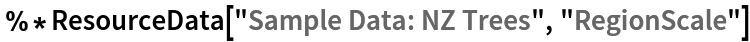Out=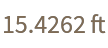Test for complete spacial randomness:

 In:=Out=Fit a Poisson point process to the data:

 In:=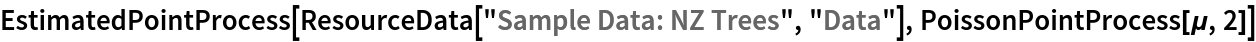Out=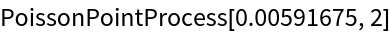Gosia Konwerska, "Sample Data: NZ Trees" from the Wolfram Data Repository (2022)